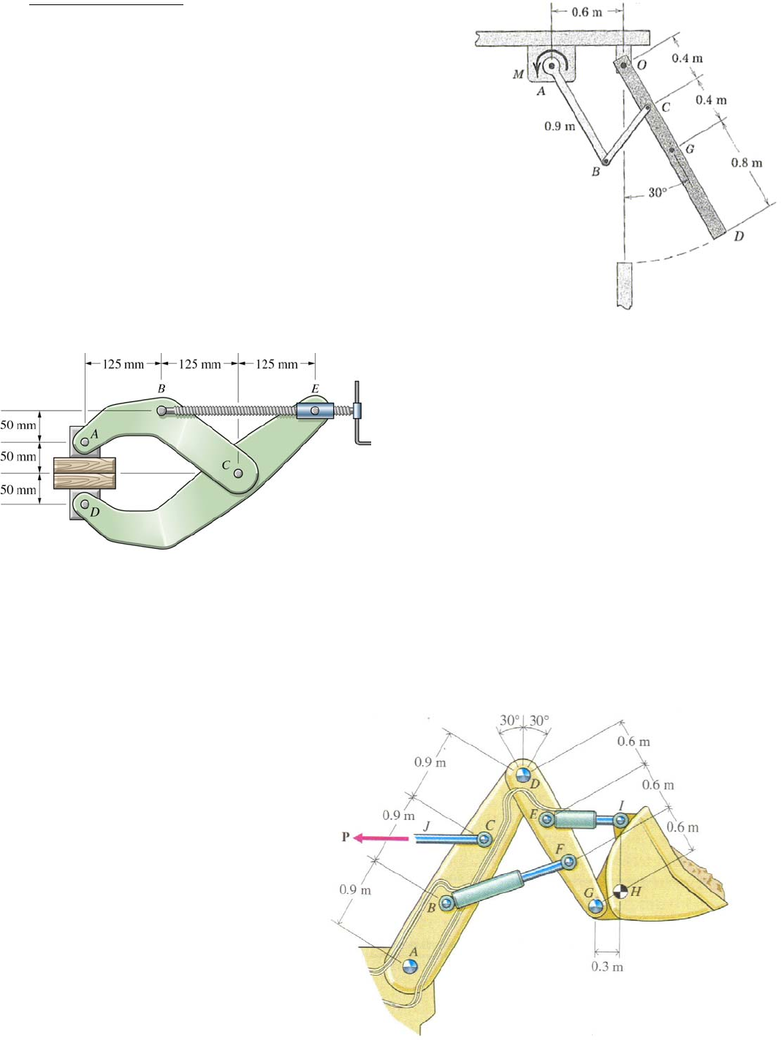# ENGG 202 Lecture Notes - Lecture 1: Bulldozer

454 views3 pagesENGG 202 Tutorial 12 April 2/3, 2013
Frames and Machines
1) The 80 kg ventilation door OD with mass center at G is
held in the equilibrium position shown (open) by means of a
moment M applied at A to the linkage mechanism. Member
AB is parallel to the door for the 30˚ position shown. All
joints are pin connections.
a) Determine the forces acting on member OCGD at O and C.
b) Determine M
ANS: CB=840.9 N (C), Ox= -528.6N, Oy=130.8N, M = 706.3 Nm
Solution Strategy: a) draw the FBD of the gate OCGD, there will be 3 unknowns
(2 at O and CB is a 2 force member), write 3 eqns of equil to solve for these
unknowns. You will need to apply some knowledge of geometry to find the angle
of member CB. B) Next draw an FBD of the member AB, there will be 2 new
unknowns at A as well as the unknown M. sum moments about A and solve for M.
2) The clamp presses two blocks of wood
together. Determine the horizontal and vertical
reactions at pin C if the blocks are pressed
together with a vertical normal force of 200 N.
ANS: Cx = 500 N, Cy = -200 N (on ABC)
Solution Strategy: draw the FBD of the upper
jaw plus upper block, there are only 3
unknowns. (force acting upward at block of
200 N, BE is a 2 force member, 2 unknowns at
C), solve.
3) The loaded bucket of the bulldozer weighs 11 kN with a centre of mass at H. The weight of
arm AD weighs 2.5 kN and acts at B, while the weight of arm DG is 1.5 kN and acts at E. The
weights of the hydraulic pistons (CJ, EI, and BF) can be neglected. Find the force P in piston CJ
and the forces in pistons BF and EI for static equilibrium. Pistons CJ and EI are horizontal.
ANS: CJ = 20.3 kN (T), BF = 11.6 kN
(C), EI = 3.18 kN (T)
Solution Strategy: draw the FBD of the
bucket, there are only 3 unknowns (2 at
G and 1 at I), write 1 eqns of equil (sum
moments about G) and solve for EI.
Next draw an FBD of arm DEFG and
the bucket H (do not detach EI), there
are 3 unknowns (2 at D and 1 at F),
write 1 eqns of equil (sum moments
Unlock document

This preview shows page 1 of the document.
Unlock all 3 pages and 3 million more documents.